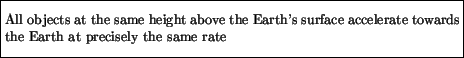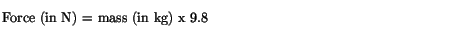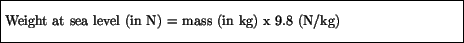Next: Questions Up: Forces and Newton's Laws Previous: Newton's 3rd Law

# Weight and Mass

The word weight was used above to denote the force with which the book pressed on the table as a consequence of the gravitational force of attraction between the Earth and the book, and Newton's third law. Here we give a more rigorous definition of the term weight. Specifically,Since forces are measured in Newtons, so is weight. An analogous definition for the weight of an object on the moon or any other planet can also be made. We thus see that the weight of an object is different on different planets, as the force of attraction would be different (this force depends on the planet's mass and radius). Even on earth, weight changes with changing altitude: the higher up you go, the less force the earth exerts on you, and hence your weight decreases. Ultimately one reaches a height where the earth exerts negligible force on you, and you experience weightlessness. We will learn more about gravity, and how it varies from place to place and planet to planet, in Chapter 6.

From the above discussion we see that mass and weight cannot strictly speaking be equivalent. Mass is the resistance of an object to a change in its motion, while weight is the gravitational force of attraction between the object and the Earth. Mass and weight even have different units. Nonetheless, the terms weight and mass are sometimes used interchangeably. For example, when we weigh an object the result is sometimes given in kilograms, and sometimes in pounds. We learn while shopping that 454 grams of cherries is the same as 1 lb of cherries. However, in the British system of units the pound'' is a unit of force that is used as the standard unit of weight (the related unit of mass is called the slug'', which is probably why the pound was adopted as the standard). The conversion to MKS units is given byNewton's second law plus a remarkable fact first discovered by Galileo can be used to provide us with a relationship between mass and weight that is implicitly used whenever something is said to weigh'' 1 kg, for example. Since force equals mass times acceleration, and weight is the force of gravity on an object we have thatThe remarkable fact realized by Galilean is that all objects accelerate towards the Earth at the same rate. You can verify this yourself by doing the following simple experiment. Hold a sheet of paper in one hand and your physics text book in the other, and drop them simultaneously from the same height above your desk. Do they hit the desk at the same time? The answer is no, but we know that the reason has to do with air resistance, which has more effect on the light piece of paper than on the heavy text book. Now if the piece of paper is just a bit smaller than the size of your textbook you can place the paper on top of the book, and drop the two together. You should find, quite dramatically, that the book and paper hit the desk at precisely the same instant. What is happening in this case is that the book is shielding the paper from the effects of air resistance, so that this experiment verifies the fact that:At sea level, this rate is 9.8 m/s2.

Going back to Newton's 2nd law, the force on an object due to gravity, at sea level, must therefore beand since this force by definition is the weight, we seeThe upshot of all this is that the weight of an object is proportional to its inertial mass and is completely independent of the object's composition. Consequently, one kilogram of anything weighs 9.8 Newtons, and we can convert'' back and forth between kilograms and Newtons using the conversion factor of 9.8 Newtons per kilogram. This however should not be viewed as a simple conversion of units like between, for example, meters and kilometers; the weight and mass measure different things, whereas meters and kilometers are both units of distance. As a check, we recall that 1 pound is equivalent to 4.45 Newtons. Converting'' 4.45 Newtons to kilograms by dividing by 9.8 Newtons per kilogram we find that 1 pound is equivalent'' to
4.45 N/ (9.8 N/kg) = .454 kg

It is important to note that there is no a priori reason for gravitational forces (i.e. weight) to be proportional to inertial mass. For example, electrical forces depend on the electrical charge of an object, and have nothing to do with inertial mass. The explanation for this riddle comes from Einstein's general theory of relativity, which we will discuss in Chapter 6.Next: Questions Up: Forces and Newton's Laws Previous: Newton's 3rd Law
modtech@theory.uwinnipeg.ca
1999-09-29